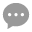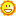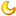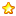# [GOM引擎] 魔界龙渊单职业版-传奇服务端源码含补丁【免费下载】1483 |18 | 2020-9-16 15:08:55 | 显示全部楼层 |阅读模式
传奇引擎: GOM引擎 » 老GOM 第三方测试登录器 » 绿盟登录器 无配套模板 服务端版本：6600 MB PC端 自定义传奇客户端(独家版本) 魔界龙渊单职业版本服务端完整,回复后免费下载

好消息：魔界龙渊单职业版-传奇服务端源码含补丁【免费下载】！！！
魔界龙渊单职业版-传奇服务端安装说明
将945Engine.dll复制并放在Mir200文件夹里面， 并在Mir200目录中的PlugList.txt文件中添加945Engine.dll即可。
魔界龙渊传奇端源游戏ESP默认配置:
主城不验证=1
脱机验证开启框=0
首次攻击怪多少分钟=61
再次攻击怪多少分钟=60
验证超时触发=0
验证取反模式=0
验证阶段人物无敌=1
开启传奇精灵=1
设置开启传奇精灵条件=1
N变量位置=47
传奇精灵提示_1=传奇精灵已准备就绪 ---- [~键] 激活自动捡物吧!
传奇精灵提示1=您不满足使用精灵服务的条件,已停止!
捡物范围=5
捡物取反模式=0
人物装备掉落不捡取=0
人物装备掉落保护时间=60000
设置捡物间隔=0
捡物间隔=2000
精灵自定义状态文本=欢迎使用传奇精灵助手允许叠加=0
移动阈值=16
攻击阈值=16
速度检测项1=1
速度检测项2=1
延迟小退=0
锁定秒数框2=5
人物挖地=1
移动全局补差值3=-30
物理全局补差值5=-30
魔法全局补差值=-60
移动智能限速=1
物理魔法智能限速=1
禁止扔物品=0
攻城行会限制=0
不卡模式短暂性允许=0
正常攻击累计=3
宝宝继承人物爆率=0
超速触发=0
多倍移动检测=0
控制移动数量=2
控制移动过滤=16
移动数量模式=0
允许云端下发插件框=1
自动同步=1
虚拟机=1
多开数量=2
是否多开=0
代理加速器=0
走位捡物框=0
允许喊话=0
物理魔法不卡=1
移动不卡=0
清除恶意进程=1
不输出M2=0
开挂触发=0
不输出日志文件=0
定向野蛮=0
开关内挂走位刺杀=0
下发流量控制二=1
下发控制二时间=8
禁止未完成加载时攻击A=0
不反挂=0兼容内挂脱机=0
转身检测=1
吃药检测=1
开启跑位攻击检测=0
开启攻击跑位检测=0
交易状态检测=1
挖地间隔检测=1
修复零血不死=1
人物刀刀命中=1
开启检测购买商品=1
开启检测非法小退A=0
移动封包检测=1
攻击封包检测=1
魔法封包检测=1
定向野蛮检测A=1

 基本介绍 默认值 时间间隔 10 最小等级 20 最大等级 500 最小元宝 20 最大元宝 5000000000 自动刷新 真

 坐沙发了哦~~谢谢分享了~~

 66666 666666

 0000000000

 8888888888666666666666666666

 zhewanyishizhenshijia

 1111111111111111111111

 正在找这个龙渊版本 谢谢分享

 您需要登录后才可以回帖 登录 | 注册传奇GM论坛账号 本版积分规则 回帖后跳转到最后一页932主题 4万积分41669